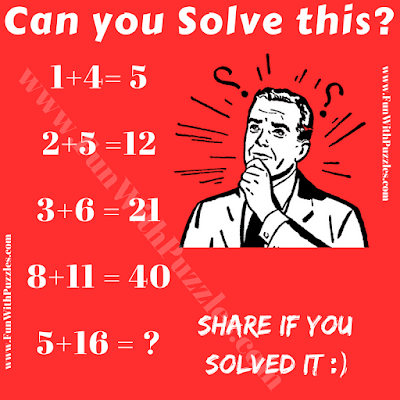This is very interesting and mind-twisting logic Math Problem for adults. In this logical puzzle, looking at the first three logical statements, one will be able to find the logical pattern in these equations. However when one comes across the 4th logical statement or logical equation, then the previous thought logic fails. Let's see if you are able to find this logical pattern in your first try and solve this Logic Math Problem?Can you solve this Logic Math Problem?

The answer to this "Logic Math Problems for Adults", can be viewed by clicking on the button. Please do give your best try before looking at the answer.

#### 1 comment:

Unknown said...

I am a Kid but I answered this My parents didn't...
Answer is 85 as you will do 16*5+5=85.